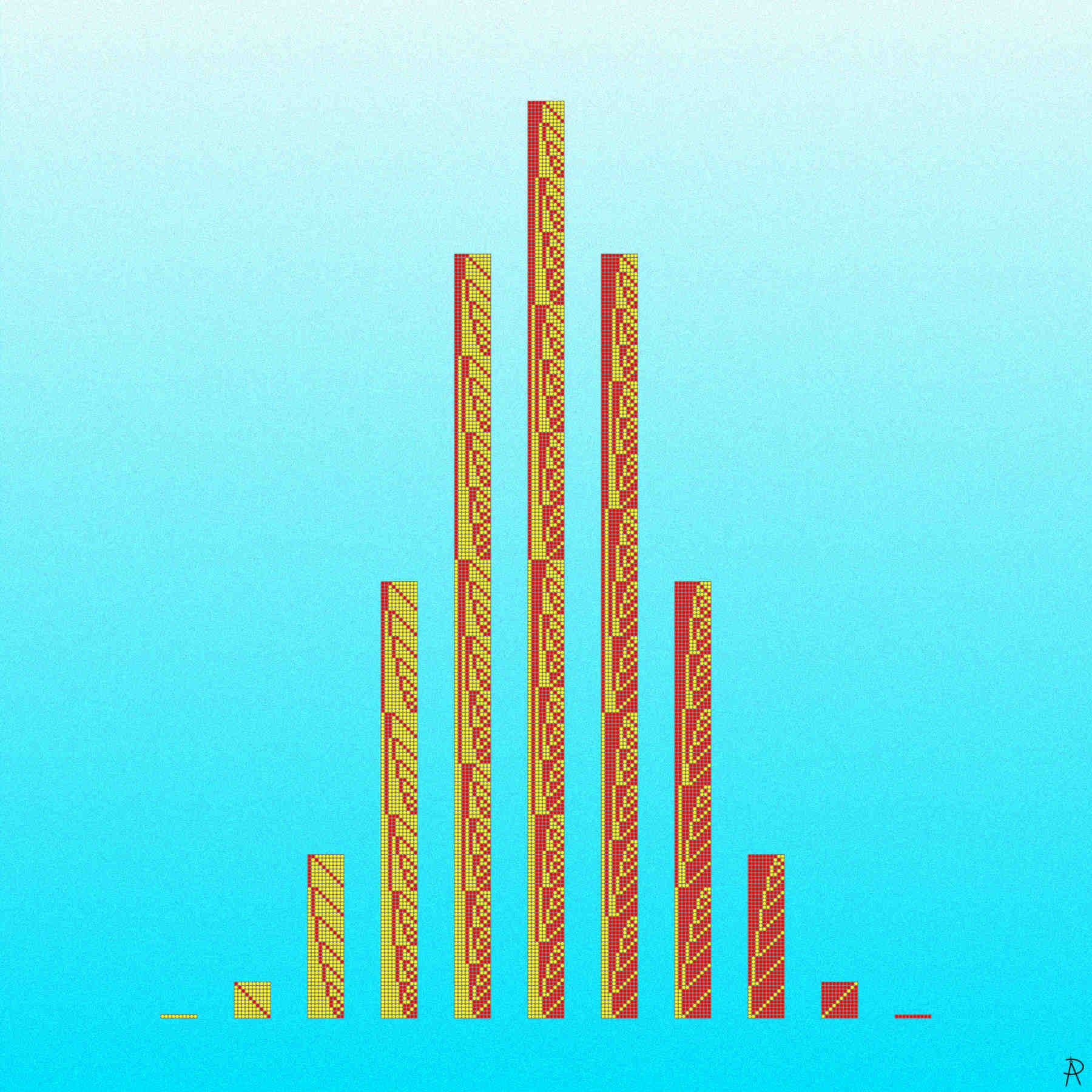Binomial Bits Digital Print 2021

All patterns of 10-bit binary patterns are shown with a one represented by a yellow dot and a zero represented with a red dot. The patterns are grouped into the 11 possibilities for the number of ones (or zeros) in a pattern. The number in each group is a binomial coefficient related the number of ways to choose $$k$$ items from a set of 10 items, resulting in a binomial distribution.

### Background and Inspiration

This piece is a blend of several key mathematical ideas: Binary numbers that are the heart of modern computers and binomial coefficients that give the number of ways of choosing $$k$$ items from a set of $$n$$ items.

Binary is used to represent every piece of information processed on modern electronic computers. Computers represent numbers, text, instructions, and other data using binary patterns. A pattern by itself carries no intrinsic meaning. Only the interpretation imparts meaning to a bit pattern. A single bit can represent just two states, but a string of $$n$$ bits can represent $$2^n$$ different patterns. Because an $$n$$-bit pattern can represent every integer from $$0$$ to $$2^n-1$$, there are $$2^{10} = 1024$$ possible ten bit patterns shown in the artwork.

The number of choosing $$k$$ items from a set of $$n$$ items is given by row $$n$$ of Pascal's triangle (see below). This artwork corresponds to $$n = 10$$. When $$k$$ is 0 or 10, there is only 1 possible pattern. When $$k$$ is 1 or 9, there are 10 possible patterns. For $$k$$ or $$10-k$$ equaling 2, 3, 4, and 5, the number of possible patterns are 45, 120, 210, and 252 respectively.

The columns in the artwork of patterns with $$k$$ ones is analogous to the bins in a Galton board with ten rows of pins. A yellow dot represents a bounce to the left and a red dot represents a bounce to the right. With ten bounces, there are $$2^{10}$$ possible bounce patterns, resulting in a binomial distribution. Galton used the Galton board to visualize the how the central limit theorem connects the binomial and normal distributions. Thus the heights of the columns show a normal bell shaped curve.